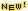Yotsanan Meemark

ยศนันต์ มีมาก
Department of Mathematics and Computer Science,

Faculty of Science, Chulalongkorn University,
Pathumwan, Bangkok 10330 Thailand

yzm101@yahoo.com  +66-2-218-5155

2015–present Chulalongkorn University (Professor)
2012–2015 Chulalongkorn University (Associate Professor)
2009–2012 Chulalongkorn University (Assistant Professor)
2006–2009 Chulalongkorn University (Lecturer)

Research Interests:
Algebraic Number Theory; Combinatorial Number Theory; Algebraic Graph Theory; Commutative Ring Theory.

Office Hours: MO 10
11,  TU 1011,  WE 910, TH 1314
Course Supplements:

 UG Linear Algebra 1/60(in Thai)| Linear Algebra II  Supplement: Practice Exam Calculus (in Thai) Calculus I | Calculus II  (in Thai)GRAD Abstract Algebra  Book: Abstract Algebra 2nd edn   Supplement: Number TheoryGrad Abst Algebra I 1/61HandOut | Grad Abst Algebra II 2/60   HandOut |

Publications:

1. Sripaisan N. and Meemark Y., Algebraic degree of spectra of Cayley hypergraphs, Discret. Appl. Math. 2022; 316 87–94.
2. Rattanakangwanwong J. and Meemark Y., Subconstituents of unitary Cayley graph of matrix algebras, Finite Fields Appl. 2022; 80: #102004.
3. Rattanakangwanwong J. and Meemark Y., Eigenvalues of zero divisor graphs of principal ideal rings, Linear Multilinear Algebra 2021 DOI:10.1080/03081087.2021.1917501
4. Sripaisan N. and Meemark Y., Approximately mutually unbiased bases by Frobenius rings, J. Syst. Sci. Complex 2020; 33 12441251.
5. Sirisuk S. and Meemark Y., Matrix graphs and MRD codes over finite principal ideal rings, Finite Fields Appl. 2020; 66: #101705.
6. Rattanakangwanwong J. and Meemark Y., Unitary Cayley graphs of matrix rings over finite commutative rings, Finite Fields Appl. 2020; 65: #101689.
7. Meemark Y. and Samart D., Mahler measures of a family of non-tempered polynomials and Boyd’s conjectures, Res. Math. Sci. 2020; 7 No.1 (20 pages).
8. Sirisuk S. and Meemark Y., Generalized symplectic graphs and generalized orthogonal graphs over finite commutative rings, Linear Multilinear Algebra 2019; 67 No.12: 2427–2450.
9. Meemark Y. and Sriwongsa S., Antiderivatives and linear differential equations using matrix, Involve, a Journal of Mathematics 2019; 12-1: 151156.
10. Thongsomnuk I. and Meemark Y., Perfect state transfer in unitary Cayley graphs and gcd-graphs, Linear Multilinear Algebra 2019; 67 No.1: 39–50.
11. Rasri A. and Meemark Y., Algebraic Cayley graphs over finite local rings, Finite Fields Appl. 2017; 48: 227–240.
12. Iamthong K. and Meemark Y., Euler quotients and wilson quotients for polynomials over finite local rings, J. Algebra Appl. 2018; 17 No.8 1850152 (12 pages).
13. Meemark Y. and Vipismakul W., A recursive formula for group determinant coefficients, J. Algebra Appl. 2018; 17 No.4 1850058 (11 pages).
14. Tocharoenirattisai I. and Meemark Y., Exponent of local ring extensions of Galois rings and digraphs of the kth power mapping, Turk. J. Math. 2017; 41 223234.
15. Meemark Y. and Sriwongsa S., Orthogonal graphs over finite commutative rings of odd characteristic, Finite Fields Appl. 2016; 40: 26–45.
16. Suntornpoch B. and Meemark Y., Cayley graphs over a finite chain ring and gcd-graphs, Bull. Aust. Math. Soc. 2016; 93: 353363.
17. Meemark Y. and Prinyasart T., A combinatorial proof of decomposition property of reduced residue systems, Involve, a Journal of Mathematics, 2016; 9-3: 361366.
18. Meemark Y. and Sriwongsa S., Perfect state transfer in unitary Cayley graphs over local rings, Trans. Comb. 2014; 3 no. 4: 43–54.
19. Meemark Y. and Puirod T., Symplectic graphs over finite commutative rings, Europ. J. Combinatorics 2014; 41: 298–307.
20. Meemark Y. and Suntornpoch B., Balanced unitary Cayley sigraphs over finite commutative rings, J. Algebra Appl. 2014; 13 No.5 1350152 (12 pages).
21. Meemark Y. and Suntornpoch B., Eigenvalues and energy of restricted unitary Cayley graphs induced from the square mapping, ScienceAsia 2013; 39 No.6: 649652.
22. Meemark Y. and Puirod T., Symplectic graphs over finite local rings, Europ. J. Combinatorics 2013; 34: 1114–1124.
23. Leela-apiradee W. and Meemark Y., Isomorphism testing for graph C_G(a,b), Notes on Number Theory and Discrete Math. 2012; 18 No.3: 20–34.
24. Meemark Y. and Wiroonsri N., The digraph of the kth power mapping of the quotient ring of polynomials over finite fields, Finite Fields Appl. 2012; 18: 179–191.
25. Meemark Y. and Wongpradit A., Permutation polynomials and elliptic curves, Notes on Number Theory and Discrete Math. 2011; 17 No.4: 1–8.
26. Kiani D., Aghaei M.M.H., Meemark Y. and Suntornpoch B., Energy of unitary Cayley graphs and gcd-graphs, Linear Algebra Appl. 2011; 435: 1336–1343.
27. Meemark Y. and Prinyasart T., On symplectic graphs modulo p^n, Discrete Math. 2011; 311: 1874–1878.
28. Meemark Y. and Maingam N., The digraph of the square mapping on quotient rings over the Gaussian integers, Int. J. Number Theory 2011; 7: 835–852.
29. Meemark Y. and Leela-apiradee W., A change of basis matrix and integrals of power of cosine, J. Statist. Plann. Inference 2011; 141: 1319–1324.
30. Meemark Y. and Sangvisut E., Near media and their representations, Int. J. Pure Appl. Math. 2010; 64: 21–30.
31. Meemark Y. and Wiroonsri N., The quadratic digraph on polynomial rings over finite fields, Finite Fields Appl. 2010; 16: 334–346.
32. Meemark Y. and Chinwarakorn S., Lerch's theorem over function fields, Integers 2010; 10: 25–30.
33. Meemark Y. and Pinthubthaworn C., Nathanson's height and the CSS conjecture for Cayley graphs, J. Math. Research 2009; 1: 3–7.
34. Meemark Y. and Thitipak T., An equivalence relation on a set of words of finite length, Europ. J. Combinatorics 2009; 30: 788–797.
35. Li W.-C. W. and Meemark Y., Hecke operators on Drinfeld cusp forms, J. Number Theory 2008; 128: 1941–1965.
36. Li W.-C. W. and Meemark Y., Ramanujan graphs on cosets of PGL_2(F_q), Finite Fields Appl. 2005; 11: 511–543.